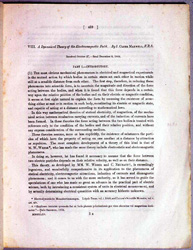﻿ The Dawn Science and Technology | Kanazawa Institute of Technology Library Center

 A dynamical theory of the electromagnetic field 1865James Clerk Maxwell(1831-1879) Maxwell, a precocious and versatile genius, researched many important problems in various fields of science some of which were the rings of Saturn, thermodynamics, the kinetic theory of gas and the theory of color, etc. in his early life. It is a marvel that all of these achievements were initiated and became fundamental contributions in each field. Among these Maxwell’s greatest achievement was the expansion of Faraday’s electromagnetic theory. Faraday, one of the greatest experimentalists in the history of science and a man of magnificent intuition, lacked the ability to express his theories mathematically. It was Maxwell who succeeded in formulating and developing Faraday’s theory of electromagnetic induction with mathematical notation.  In the two papers which preceded this work, Maxwell investigated the theory of the line of force and attempted to give it mathematical expression. First, using a hydrodynamical analogy, he regarded the line of magnetic force as the line of a fluid’s stream and expressed this mathematically, but electromagnetic induction proved incapable of being explained by this analogy. Maxwell then came up with a new idea which enabled him to explain the vertiginous quality of magnetism and the generation of electric current that corresponds to the change of intensity in magnetism. Assuming that the line of magnetic force was like the rotating fine cylinder in screwed vortex motion along the longitudinal axis of the cylinder, he devised infinitesimal spherical particles between the cylinders which would make possible the rotation of the cylinders. That is, the locomotion of these particles which were at right angles to the axis of the vortex was the electric current. Although this highly sophisticated, mechanistic analogy was a complicated, awkward system, it completely explained electro-magnetic induction.  In this paper, Maxwell gave exquisite, mathematical formulation to the above mentioned model with vector analysis which was developed by Hamilton. He reasoned out several four-dimensional equations which were called Maxwell’s relations based on the concept of “field”. These purely mathematical deductions inevitably led him to forecast the electromagnetic wave as a logical consequence. He also demonstrated that the velocity of a propagation of such a wave is equal to the velocity of light. That is, he made known the fundamental principle that light is the electromagnetic wave. Thus, Maxwell established a comprehensive theory of the electromagnetic field which provided a firm foundation for Einstein.